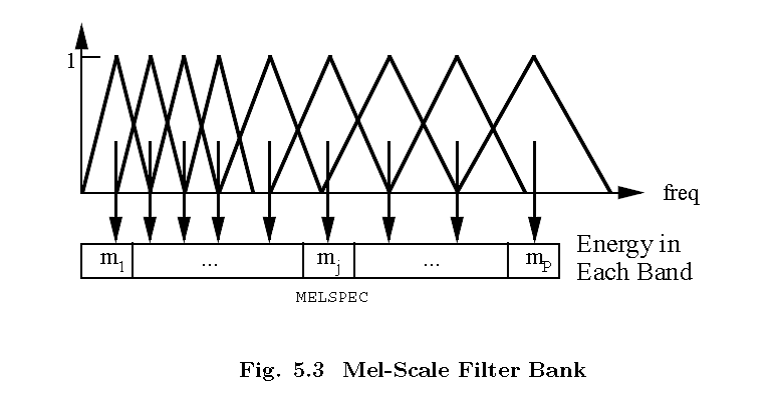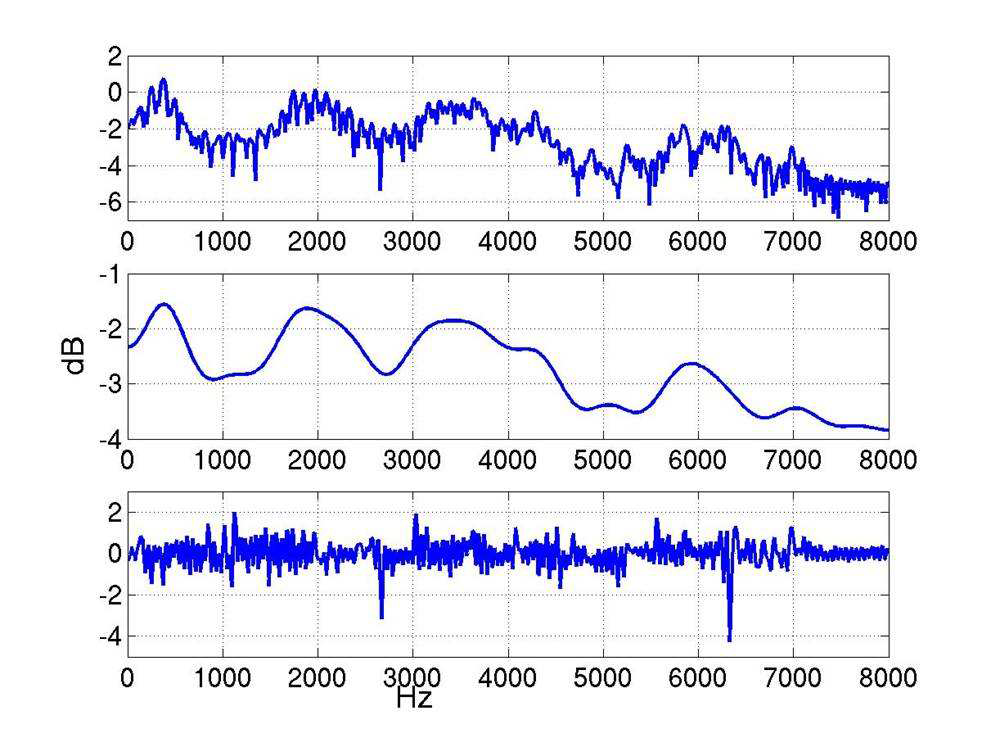# 声学特征（二） MFCC特征原理

## 基本含义

MFCC是Mel-Frequency Cepstral Coefficients的缩写，顾名思义MFCC特征提取包含两个关键步骤：转化到梅尔频率，然后进行倒谱分析

### 梅尔频率

m=2595log10(1+f700)### 倒谱分析X(k)=DFT(x(n))

X(k)=H(k)E(k)

x(n)=h(n)e(n)

log(X(k))=log(H(k))+log(E(k))

IDFT(log(X(k)))=IDFT(log(H(k)))+IDFT(log(E(k)))

x(n)=h(n)+e(n)

## 基本流程

### 预加重

1. 目的
为了消除发声过程中，声带和嘴唇造成的效应，来补偿语音信号受到发音系统所压抑的高频部分。并且能突显高频的共振峰。
简单理解就是在频域上面都乘以一个系数，这个系数跟频率成正相关，所以高频的幅值会有所提升。实际上就是通过了一个H(z)=1kz1$H(z)=1-kz^{-1}$高通滤波器。
2. 实现
sn=snksn1

### 加窗

1. 目的
用于平滑信号，使用汉明窗加以平滑的话，相比于矩形窗函数，会减弱FFT以后旁瓣大小以及频谱泄露。
2. 实现
使用汉明窗对信号进行加窗处理
sn={0.540.46cos(2π(n1)N1)}sn

### 频域转换

1. 目的
将时域信号转化到频域进行后续的频率分析
2. 实现
幅度谱：
Si(k)=n=1Nsi(n)ej2πkn/N1kK

功率谱：
Pi(k)=1N|Si(k)2|

### 使用梅尔刻度滤波器组过滤

1. 目的
因为频域信号有很多冗余，滤波器组可以对频域的幅值进行精简，每一个频段用一个值来表示。
2. 实现
对于FFT得到的幅度谱，分别跟每一个滤波器进行频率相乘累加，得到的值即为该帧数据在在该滤波器对应频段的能量值。如果滤波器的个数为22，那么此时应该得到22个能量值

### 离散余弦变换

DCT和DFT类似，但是只使用实数，不涉及复数运算。信号经过DCT变换以后，能量会集中到低频部分，可以用于图像压缩。
1. 目的

2. 实现

ci=2Nj=1Nmjcos(πiN(j0.5))

### 差分

1. 目的
由于语音信号是时域连续的，分帧提取的特征信息只反应了本帧语音的特性，为了使特征更能体现时域连续性，可以在特征维度增加前后帧信息的维度。常用的是一阶差分和二阶差分。
2. 实现
dt=Θθ=1θ(ct+θctθ)2Θθ=1θ2

## MFSC和MFCC

- 对特征进行了去相关和压缩，更适合Diagonal covariance GMMs这种建模方式。
- MFCC数据量少。

- MFSC具有相关性，表现在频谱上面的较为平滑
- MFSC具有更高的维度

## 参考文献

©️2019 CSDN 皮肤主题: 大白 设计师: CSDN官方博客